# SQUARE ROOTS PRESENTED BY MR LAWS 8 TH

• Slides: 13
Download presentation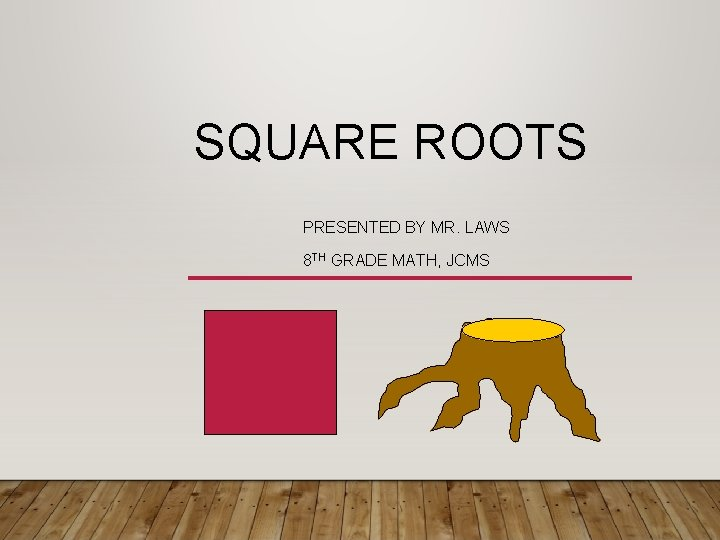SQUARE ROOTS PRESENTED BY MR. LAWS 8 TH GRADE MATH, JCMSGOAL/OBJECTIVE • 8. EE. 2 –Use square and cube roots symbols to represent solutions to equations of the form x 2=p and x 3=p, where p is a positive rational number. • Evaluate square roots of small perfect squares and cube roots of small perfect cubes. Know that is irrational.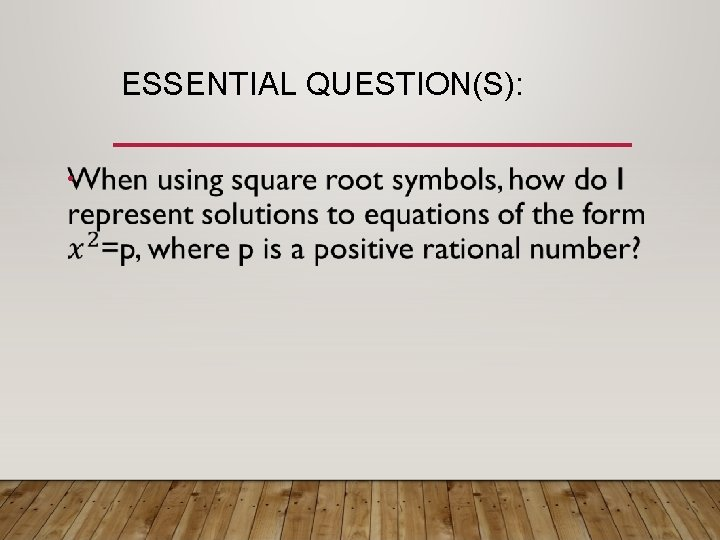ESSENTIAL QUESTION(S): •WHAT IS A SQUARE ROOT? index Radical sign Square Root 16 = 4 Radicand • Radical sign indicates a root. • Radicand is the number under the radical sign. • Index displays the root of an expression. 42 = 16 1. The square root of a number is a number when multiplied by itself two times equals the radicand.SQUARE ROOTS 2. The inverse operation of squaring is finding the square root. 3. A square root of a number is any number that can be multiplied by itself to get the original number. • For example: 3 is the square root of 9 because 3 x 3 = 9 • Also, the square root of 9 can be -3 because (-3) x (-3) = Example 9: Positive Square Root Negative Square RootWHAT ARE PERFECT SQUARES? 4. Perfect Squares are square roots when multiplied by itself 2 times will equal an integer(number). 5. Perfect Squares are rational numbers. 6. There are 20 perfect squares that you need to know.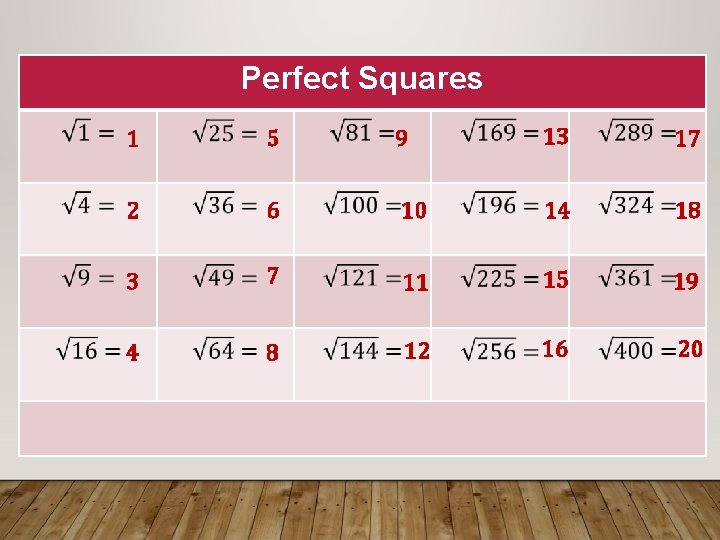Perfect Squares 1 5 9 13 17 2 6 10 14 18 3 7 11 15 19 4 8 12 16 20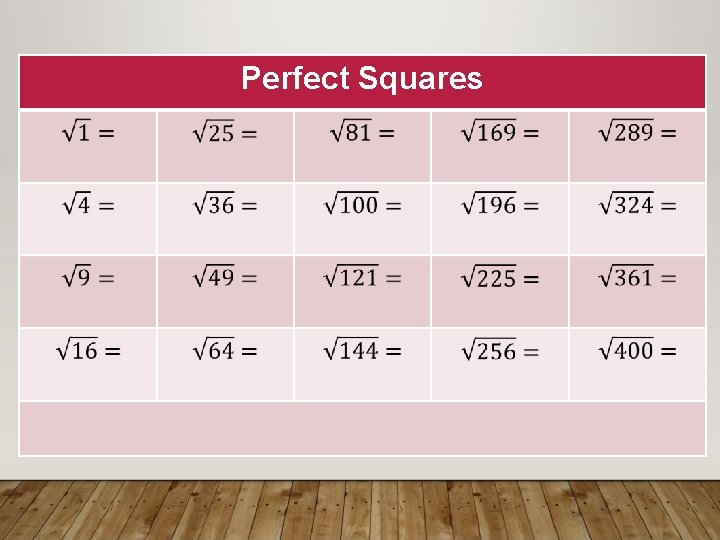Perfect Squares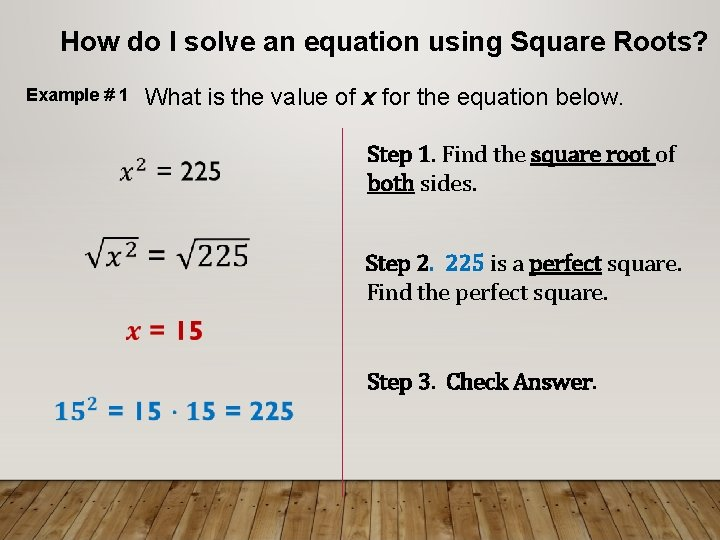How do I solve an equation using Square Roots? What is the value of x for the equation below. Example # 1 Step 1. Find the square root of both sides. Step 2. 225 is a perfect square. Find the perfect square. Step 3. Check Answer.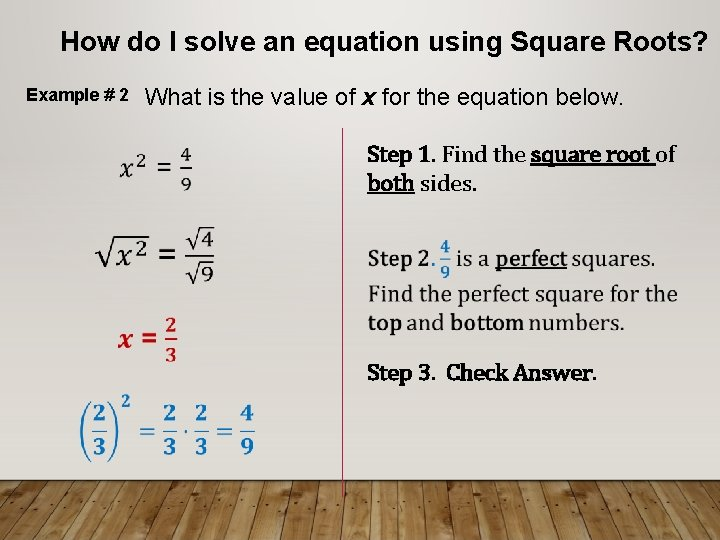How do I solve an equation using Square Roots? Example # 2 What is the value of x for the equation below. Step 1. Find the square root of both sides. Step 3. Check Answer.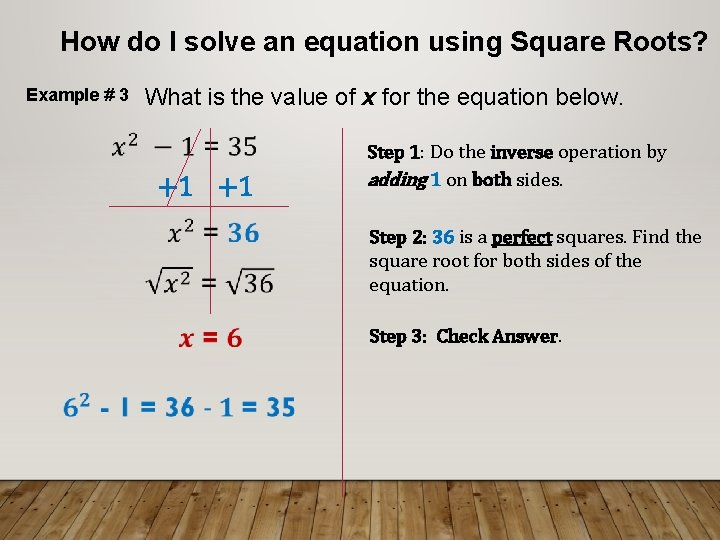How do I solve an equation using Square Roots? Example # 3 What is the value of x for the equation below. +1 +1 Step 2: 36 is a perfect squares. Find the square root for both sides of the equation. Step 1: Do the inverse operation by adding 1 on both sides. Step 3: Check Answer.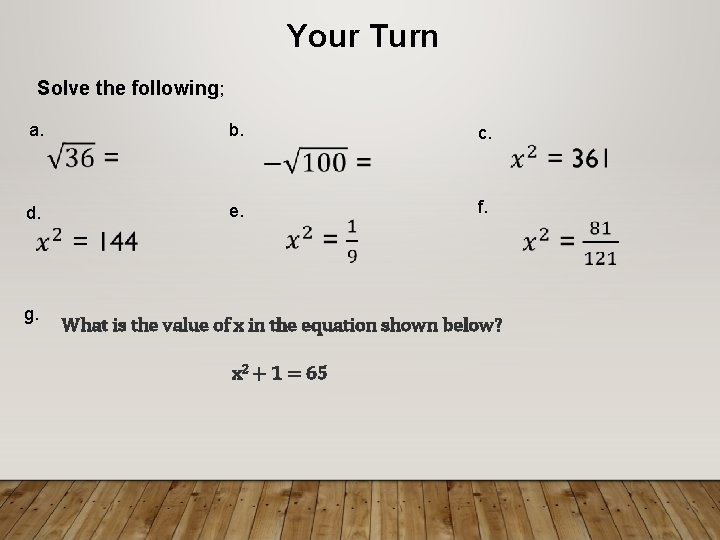Your Turn Solve the following; a. b. g. e. d. c. f. What is the value of x in the equation shown below? x 2 + 1 = 65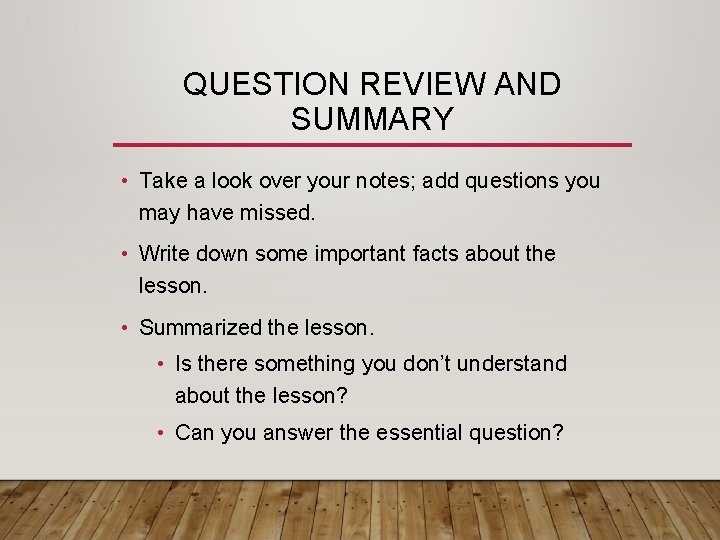QUESTION REVIEW AND SUMMARY • Take a look over your notes; add questions you may have missed. • Write down some important facts about the lesson. • Summarized the lesson. • Is there something you don’t understand about the lesson? • Can you answer the essential question?